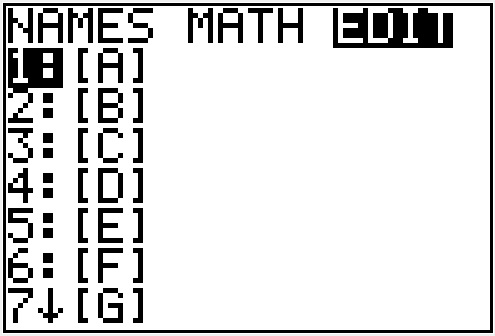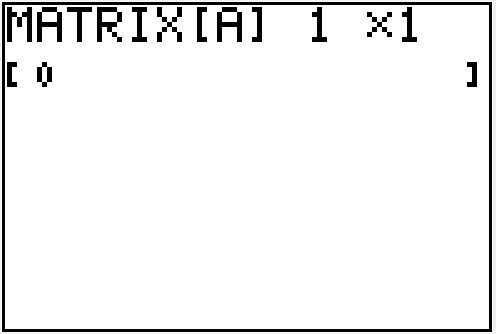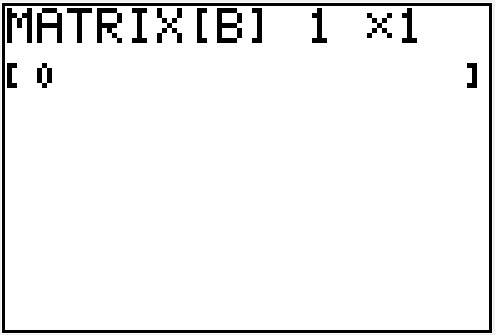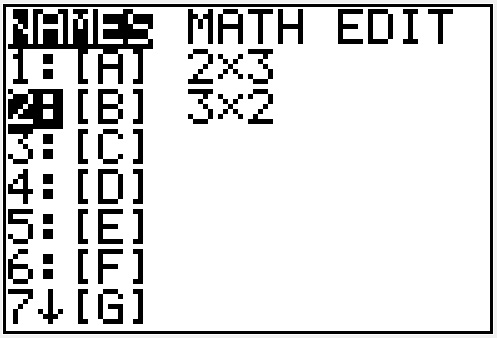﻿ Multiplying matrices with the TI83 or TI84 calculator - MathBootCamps

# Multiplying matrices with the TI83 or TI84 calculator

Graphing calculators such as the TI83 and TI84 are able to do many different operations with matrices, including multiplication. Here, we will go over the steps needed to multiply two matrices in this type of calculator using the following example.

## Step-by-step with an example

Find the product of the matrices.

$$AB=\left[\begin{array}{ccc}-1 & 5 & -2\\ 3 & 7 & 7\\\end{array}\right]\left[\begin{array}{cc}4 & 0\\ 1 & 1\\ 3 & 9\end{array}\right]$$

### Step 1: Enter the first matrix into the calculator

To enter a matrix, press [2ND] and $$\left[x^{-1}\right]$$.

(Note: some older models of the TI83 calculators have a MATRIX button)Use the right arrow key to go to the EDIT menu.Press enter to select matrix A.Type in the size of the matrix and the values by typing each number and pressing [ENTER]. Note that the first matrix is a 2 x 3 matrix (rows by columns).

$$A=\left[\begin{array}{ccc}-1 & 5 & -2\\ 3 & 7 & 7\\\end{array}\right]$$### Step 2: Enter the second matrix into the calculator

Press [2ND] and $$\left[x^{-1}\right]$$.Press the right arrow key to go to the EDIT menu.Press  or highlight 2. [B] and press [ENTER].Type in the size of the matrix and the values by typing each number and pressing [ENTER]. Note that the second matrix from our example is a 3 x 2 matrix (rows by columns).

$$\left[\begin{array}{cc}4 & 0\\ 1 & 1\\ 3 & 9\end{array}\right]$$### Step 3: Press [2ND] and [MODE] to quit out of the matrix screen

This will take you to a blank screen. If you skip this step, your calculator may (depending on where your cursor is) try to put matrix A inside of matrix B, causing an error.

### Step 4: Select matrix A and matrix B in the NAMES menu to find the product

From the blank screen, press [2ND] and $$\left[x^{-1}\right]$$ and stay at the NAMES menu.Press [ENTER] to select matrix [A].To select matrix [B], go back into the matrix menu by pressing [2ND] and $$\left[x^{-1}\right]$$.Press  or highlight 2. [B] and press [ENTER].Press [ENTER] to multiply the matrices.\begin{align} AB &=\left[\begin{array}{ccc}-1 & 5 & -2\\ 3 & 7 & 7\\\end{array}\right]\left[\begin{array}{cc}4 & 0\\ 1 & 1\\ 3 & 9\end{array}\right] \\ &=\boxed{\left[\begin{array}{cc}-5 & -13\\ 40 & 70\\\end{array}\right]}\end{align}

## Common error: DIM MISMATCH

Suppose that you go through these steps and end up with the following screen.In this case, you should check two things:

1. Did you enter the correct matrix information and did you select the correct matrices to multiply?
2. Is the product defined?

If you entered the matrices correctly, then this error means that the product is undefined. Specifically, if you write out the sizes of the matrices, the inner numbers must match. In the example above, we had a 2 x 3 and a 3 x 2. Notice that the numbers on the inside match, so the product was defined and we were able to find an answer.

However, the product of a 2 x 2 and a 3 x 2 would be undefined since the inner numbers do not match. You would simply write “Undefined” as your answer if you were working a problem like this on an exam or quiz.

When is matrix multiplication defined?

## Continue your study of matrices and the TI83 / 84 calculator